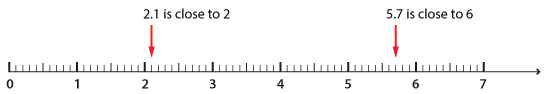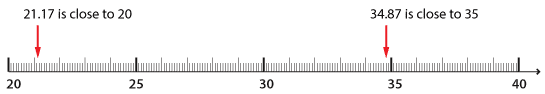#### Checking the reasonableness of answers

Sometimes it can be easy to make a simple error in calculations involving addition. It is important to use knowledge of place value to check whether your answer is reasonable.

With a simple calculation such as 5.7 + 2.1, we round off each number to the nearest whole number and then add to give an estimate. 5.7 is rounded to 6 and 2.1 is rounded to 2. So our estimate is 6 + 2 = 8.

The use of a number line helps to make this more evident:Detailed description

When you do the calculation, you find you are correct.

 5 . 7 + 2 . 1 7 . 8

If the numbers being added involve hundredths, the number line can clearly demonstrate how to round to the nearest whole number:Detailed description

34.87 + 21.17 =

34.87 is rounded to 35 and 21.17 is rounded to 21.

35 + 21 is equal to 56.

When we do the calculation, we can see that the estimate is close to the answer:

 3 4 . 8 7 + 2 $$1_1$$ . $$1_1$$ 7 5 6 . 0 4